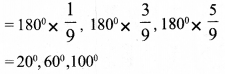# Kerala Syllabus 9th Standard Maths Solutions Chapter 12 Proportion

## Kerala State Syllabus 9th Standard Maths Solutions Chapter 12 Proportion

### Proportion Textual Questions and Answers

Textbook Page No. 182

Hss Live Guru 9th Maths Kerala Syllabus Question 1.
A person invests 10000 rupees and 15000 rupees in two different schemes. After one year, he got 900 rupees as interest for the first amount and 1200 rupees as interest for the second amount.
i. Are the interests proportional to the investments?
ii. What is the ratio of the interest to the amount invested in the first scheme? What about the second?.
iii. What is the annual rate of interest in the first scheme? And in the second?
i. The ratio between the amounts invested = 10000:15000 = 2 : 3
The ratio between the interests = 900 : 1200 = 3 : 4
Since the ratios are different. So, interests will be not proportional to the investments.

ii. The ratio between the amount invested and interest in scheme 1
= 10000 : 900= 100 : 9
The ratio between the amount invested and interest in scheme 2
= 15000 : 1200 = 25 : 2

iii. Rate of interest in the first scheme
= 900/10000 × 100 = 9%
Rate of interest in the second scheme =
1200/15000 × 100 = 8%

Make use of this free RSD calculator online that is specifically designed to calculate relative deviation of a data set.

Kerala State Class 9 Maths Solutions Question 2.
The area of A0 paper is one square metre. Calculate the lengths of the sides of A4 paper correct to a millimetre, using a calculator.
The area of A1 paper is half of the area of A0 paper. The area of A2 paper is half of the area of A1 paper. Let con-sider the area of the A0 paper be 1 square metre, the area of A1 paper is
1/2 square metre , the area of A2 paper is 1/4 square metre , the area of A3 paper is 1/8 square metre , and the area of A4 paper is 1/16 square metre ,
If the length of A4 paper is √2 x and breadth x
length of A4 paper : breadth = √2 : 1
Area of A4 paper = length x breadth
√2x × x = √2x2
This is 1/16 m2, therefore √2 x2 = 1/16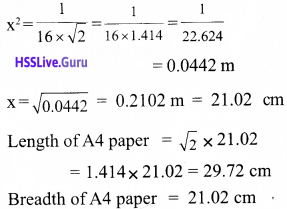Hsslive Guru 9th Maths Kerala Syllabus Question 3.
In calcium carbonate, the masses of calcium, carbon and oxygen are in the ratio 10 : 3: 12 . When 150 grams of a compound was analysed, it was found to contain 60 grams of calcium, 20 grams of carbon and 70 grams of oxygen. Is it calcium carbonate?
The ratio between calcium, carbon and oxygen in calcium carbonate = 10 : 3: 12 The ratio of calcium, carbon and oxygen in the given compound = 60 : 20: 70 = 6 : 2: 7
Since this ratio is not equal to the ratio of calcium, carbon and oxygen in calcium carbonate, it is not calcium carbonate.

Textbook Page No. 185

Hss Live 9 Maths Kerala Syllabus Question 1.
For each pair of quantities given below, check whether the first is proportional to the second. For proportional quantities, calculate the constant of proportionality.
i. Perimeter and radius of circles.
ii. Area and radius of circles.
iii. The distance travelled and the number of rotations of a circular ring moving along a line.
iv. The interest got in a year and the amount deposited in a scheme in which interest is compounded annually.
v. The volume of water poured into a hollow prism and the height of the water level.
i. The perimeter of a circle is n times its diameter. That is 2n times the radius.
∴Perimeter = 2πr
∴ The perimeter and radius are proportional.
The constant of proportionality is 2π

ii. The area of a circle is π times the square of the radius.
∴ Area = πr2
∴ Area and radius are not proportional.

iii. When the ring rotates once, the distance travelled is equal to its perimeter. When it rotates twice the distance travelled is twice its perimeter. When it rotates ‘n’ times, the distance travelled is ‘n ‘ times the perimeter of the ring.

iv. If the amount deposited is P and the rate of interest is R.
Annual interest = I = PNR,
I = P × R (N = 1)
The amount and interest are propor-tional.
Constant of proportionality is rate of interest, R.

v. Volume of a hollow prism
= base × height
Volume, of water and height of water level, are proportional. Constant of proportionality is the base area.

Kerala Syllabus 9th Standard Maths Notes Question 2.
During rainfall, the volume of water falling in each square metre may be considered equal.
i. Prove that the volume of water falling in a region is proportional to the area of the region.
ii. Explain why the heights of rainwater collected in different sized hollow prisms kept near one another are equal.
i. The volume of water falling in each square metre are equal.
Let the volume of the rain falling on the 1 square metre be k The volume of the rain falling on the 2 square metre = 2k
The volume of the rain falling on the 3 square metre = 3k
The volume of the rain falling on the x square metre y = kx Here x and y are proportional.

ii. Let x be the base area of the vessel and h be the height of the water collected, then the volume of water y = xh.
y/x =h
The volume of water collected is different in hollow prisms having different base area.

But $$\frac { volume }{ Area }$$ is always equal to the height of water level. So the height at which rainwater collected is same.

Hsslive Guru Maths 9th Kerala Syllabus Question 3.
When a weight is suspended by a spring, the extension is proportional to the weight. Explain how this can be used to mark weights on a spring balance.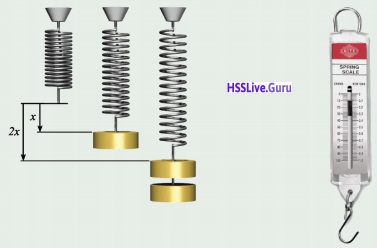Mark the pointer when no weight is hanging on it. Then mark the point when a constant weight is hanging on it. For example when we hang 1 kg on it a 2 cm extension is made. So we mark 1, 2, 3, 4 from the points marked first, i.e.,
2, 4, 6 and 8.
These distances are divided into 10 equal parts, then we can mark the points 1.1 kg and 2.4 kg etc.

Hss Live Guru 9 Maths Kerala Syllabus Question 4.
In the angle shown below, for different points on the slanted line, as the distance from the vertex of the angle changes, the height from the horizontal line also changes.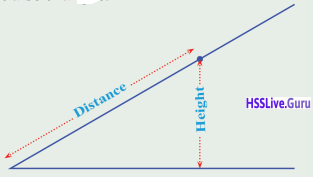i. In the picture, perpendicular to the horizontal lines are drawn from points on the slanted line.
Δ ABP, Δ ACQ, Δ ADRand Δ AES are similar triangles. (Because these are a right-angled triangle with is common to all)
∴ Ratio of their sides are equal. That is sides are proportional.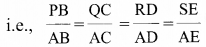Let k be the constant of proportionality
PB = k × AB, QC = k × AC
RD = k × AD, SE = k × AE ,
i.e., the change in height is proportional to the distance.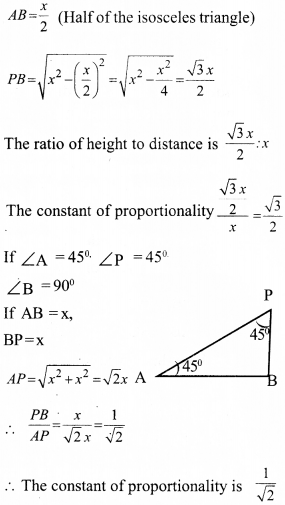An online geometric sequence calculator helps you to find geometric Sequence, first term, common ratio calculator, and the number of terms.

Textbook Page No. 189

Class 9 Maths Chapter 12 Kerala Syllabus Question 1.
i. Prove that for equilateral triangles, area is proportional to the square of the length of aside. What is the constant of proportionality?
ii For squares, is area proportional to square of the length of a side? If so, what is the constant of proportionality?
i. Δ ABC is an equilateral triangle
Consider their sides are ‘a’,
Draw a perpendicular line CD to AB
from C. In right-angled triangle ADC, according to the Pythagoras theorem AD2 + DC2 = AC2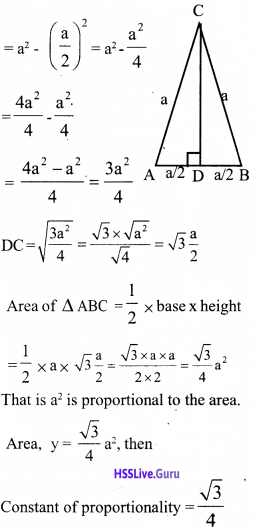ii. If x be the one side of a square then its area y = x2
Area of the square is proportional to the square of their sides.
Constant of proportionality = 1

Hsslive Guru Class 9 Maths Kerala Syllabus Question 2.
In rectangles of area one square metre, as the length of one side changes, so does the length of the other side. Write the relation between the lengths as an algebraic equation. How do we say this in the language of proportions?
Area of the rectangle is the sum product of length and breadth.
Let × be the length and y be the breadth, then
x × y = 1 m2x = 1/y = m
Example when y = 3 m
x = 1/3 meter
when y = 4 m
x = 1/4 meter
The algebraic equation is x = 1/y
i.e., length of rectangle is proportional to the reciprocal of the breadth, i.e., length of rectangle is proportional to its breadth.

Chapter 12 Maths Class 9 Kerala Syllabus Question 3.
In triangles of the same area, how do we say the relation between the length of the longest side and the length of the perpendicular from the opposite vertex? What if we take the length of the shortest side instead?
Let ‘a’ be the large side, h be the length of perpendicular from opposite vertices, ‘A’ be the area, then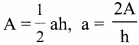Length of larger side is inversely proportional to the length of perpendicular from the opposite vertex.
i.e., The length of small side is inversely proportional to the length of perpendicular line from the vertex of small side.

Hss Live Guru Class 9 Maths Kerala Syllabus Question 4.
In regular polygons, what is the relation between the number of sides and the degree measure of an outer angle? Can it be stated in terms of proportion?
The sum of the exterior angles of all polygon is 360°. If ‘n’ is the number of sides.
Measure of an exterior angle = $$\frac{\text { Sum of exterior angles }}{\text { No. of sides }}$$
One outer angle = 360/n
(n = number of sides)
If the measure of an exterior angle is ‘x’.
x= $$\frac{1}{n} \times 360^{0}$$
One outer angle and number of sides are inversely proportional. The constant of proportionality is 1/n.

Hsslive Guru Maths Kerala Syllabus 9th Question 5.
A fixed volume of water is to flow into a rectangular water tank. The rate of flow can be changed by using different pipes. Write the relations between the following quantities as an algebraic equation and in terms of proportions.
i. The rate of water flow and the height of the water level.
ii The rate of water flow and the time taken to fill the tank.
i. Let x be the rate of water flowing, y be the height of water in the tank and A be the base area of the tank, then
x = Ay
Height of the water level in the tank is proportional to the rate of the water flowing.

ii. If C is the volume of the tank, V be the volume of water flowing per second, the volume of water in ‘t’ second is given by
C = V × t
$$V=\frac{C}{t}=C \times \frac{1}{t}$$
That is the rate of water flow and the time taken for filling the tank are inversely proportional. C is the constant of proportionality.

### Proportion Exam oriented Questions and Answers

Hsslive 9th Maths Kerala Syllabus Question 1.
the weight of 6 spheres of same size made of the same metal is 14 kg. When 9 more spheres are added the weight is 35 kg. Check whether the number of spheres and their weights are proportional.
Weight of 6 spheres = 14kg
Ratio of number and weight = 6 : 14 = 3 : 7
Total number of sphers are added = 15
Total weight = 35kg
Ration of number and weight = 15 : 35 = 3 : 7
Since the ratios are equal, the number of sphers and their weights are propor-tional.

Question 2.
Raghu invested Rs. 60000 and Nazar Rs. 100000 and started a business. Within one month a profit of Rs. 4800 was obtained. Raghu took 1800 and Nazar took Rs. 3000 out of the profit obtained. What is the ratio of the investment? Is the investment and the profit divided proportionally?
Ratio of investments = 60000 : 100000 = 6 : 10 = 3 : 5
Ratio of profit divided
1800 : 3000 = 18 : 30 = 3 : 5
Ratio of investments and Ratio of profit divided are equal. Hence they are proportional.

Question 3.
The two sides of a triangle having perimeter 10 m are 2$$\frac { 1 }{ 2 }$$ m and 3 $$\frac { 1 }{ 2 }$$ m.
What is the ratio of the length of the three sides of triangles?
Perimeter of triangle = 10 m
First side = 3$$\frac { 1 }{ 2 }$$ m = $$\frac { 5 }{ 2 }$$ m
Second side = 3$$\frac { 1 }{ 2 }$$ m = $$\frac { 7 }{ 2 }$$ m
Third side = 4 m Ratio of three sides of a triangle
$$=\frac{5}{2}: \frac{7}{2}: 4 = 5: 7: 8$$

Question 4.
150 litres of water is flowing through a pipe in 6 minutes. If 200 litres of water flows through it in 8 minutes check whether the quantity of water and time of flow are proportional ?
Quantity of water flowing in 6 minutes = 150 litres
Ratio between the quantity of water and timeofflow= 150: 6 = 25: 1
Quantity of water flowing in 8 minutes = 200 litres
Ratio between quantity of water and times of flow = 200 : 8 = 25: 1
Since the radios are equal, the amount of water flowing and the time of flow are proportional.

Question 5.
Unniyappam was made using 1 kg rice, 250 g plantain and 750g jaggery. Find the ratio between the ingredients.
Rice = 1
kg= 1000g
Plantain = 250 g, Jaggery = 750 g.
Ratio between the ingredients
= 1000 : 250 : 750 = 4 : 1 : 3

Question 6.
Sathyan got Rs. 500 after working for 6 hours. Gopi got Rs. 400 after working for 4$$\frac { 1 }{ 2 }$$ hours. Are the wages obtained proportional to the working time ?
Ratio of working hours = 6 : 4 $$\frac { 1 }{ 2 }$$
= 12 : 9 = 4 : 3
Ratio of wages = 800 : 600 = 8 : 6 = 4 : 3
Since the ratios are equal, the working hours are proportional to the wages.

Question 7.
Are the length and breadth of a square having same perimeter inversely proportional?
for a square the perimeter is 20 cm. so,
Let x be the length and y be the breadth then possible values of x and y are
x — y
9 — 1
8 — 2
7 — 3
Here xy is not a constant term i.e. the changes in the x and y is not in the firm of xy = kept
hence length and breadth are not in inversely proportional.

Question 8.
The weight of an object having mass 5 kg is 49 Newton. The weight of another object having mass 15 kg is 147 Newton. Check whether the mass and weight are proportional ? What is the constant of proportionality? What is the weight of an object having mass 8 kg ?
Weight of 5 kg object = 49 N
Ratio between mass and weight = 5 : 49
Weight of 15 kg massed object = 147N
Ratio between mass and weight = 15:147 = 5:49
Mass and weight are proportional.
Weight of 8 kg massed object = 8 × 9.8 = 78.4NConstant of proportionality = 9.8

Question 9.
The perimeter of a triangle is 60 cm. Sides are in the ratio 3: 4: 5. Then j find the length of the sides.
Ratio of sides = 3 : 4 : 5
Therefore, the sides are the $$\frac { 3 }{ 12 }$$, $$\frac { 4 }{ 12 }$$ and $$\frac { 5 }{ 12 }$$ part of the perimeter .
The perimeter is 60 cm, then the length of sides are$$60 \times \frac{5}{12}=25 \mathrm{cm}$$

Question 10.
Will the dye which contains 10 L blue colour and 15 L white colour and another dye which contain 12L blue colour and 17 L white colour have j the same colour? Why?
In the first dye , blue colour: white colour = 10 : 15 = 2 : 3
In the second dye, blue colour: white colour = 12: 17
The ratios are not same. Hence the both will not have same colour.

Question 11.
The face perimeter of some vessels in the shape of square prisms are equal. 12 litres of water can be filled in the vessel having height 15 cm. 16 litres of water can be filled in the vessel having height 20 cm. Check whether the volumes of the vessel and height are proportional. What is the constant of proportionality?
Since the face perimeters are equal,
Volume = face perimeter × height
Volume of the vessel having height 15 cm = 12 litres.
Ratio of height to volume =15 : 12 = 5 : 4 Volume of the vessel having height 20 cm = 16 litres
Ratio of height to volume =20 : 16 = 5 : 4 Since the ratios are equal, height and volume are proportional.
$$\frac { volume }{ height }$$ = $$\frac { 4 }{ 5 }$$ constant of proportionality
Volume of the vessel having height 35 cm
= 35 × $$\frac { 4 }{ 5 }$$ = 28 liters.

Question 12.
The ratio of carbon, sulphur and potassium nitrate to make gun powder is 3 : 2: 1. How much quantity of each is required to make 1.2 kg of gun powder ?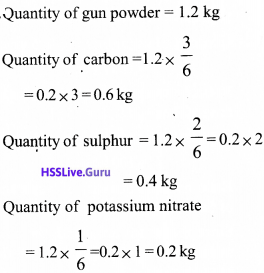Question 13.
Raju got Rs. 400 after working for 8 hours. Damu worked for 6 hours and got Rs. 300. Are the wages obtained proportional to the work time?
Ramu working hours = 8 hour
Wages Raju obtained = Rs. 400
Damu working hours = 6 hour
Wages obtained by Damu = Rs. 300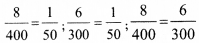∴ Wages obtained are proportional to the work time.

Question 14.
150 L of water flows through a pipe for 6 minutes. 200 L of water flows for 8 minutes through the same pipe. Are the time and amount of water flowing proportional?
Ratio of quantity of water
= 150 : 200 = 15 : 20 = 3 : 4
Ratio oftime =6 : 8 = 3 : 4
The ratios are same , hence they are proportional.

Question 15.
A car with 5L of petrol travels a distance of 75 km. What is the proportionality constant between the distance travelled and the quantity of petrol? How much petrol is needed for travelling 180 km?The angles of the traingle are $$\frac { 1 }{ 9 }$$, $$\frac { 3 }{ 9 }$$ and $$\frac { 5 }{ 9 }$$